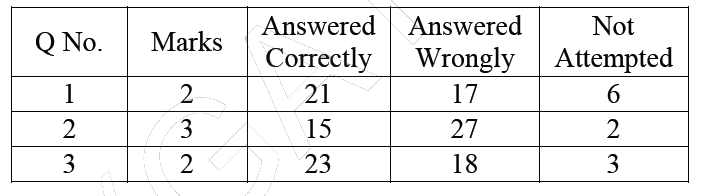GeeksforGeeks App
Open AppBrowser
Continue

# GATE | GATE-CS-2014-(Set-3) | Question 65

The table below has question-wise data on the performance of students in an examination. The marks for each question are also listed. There is no negative or partial marking in the examination.What is the average of the marks obtained by the class in the examination
(A) 1.34
(B) 1.74
(C) 3.02
(D) 3.91

Explanation:

```As you can see from each row that the total number of
students in the class are 44.

Now we have to find the total number of marks obtained
by the class.

Marks are given for correct answers only.
No negative or partial marking.

For 1st question total marks obtained are : 2*21 = 42
For 2nd question total marks obtained are : 3*15 = 45
For 3rd question total marks obtained are : 2*23 = 46

hence total marks obtained by the class are = 42+45+46 = 133

Now, Average marks obtained = Total Marks/Total no of students
= 133/44
= 3.02